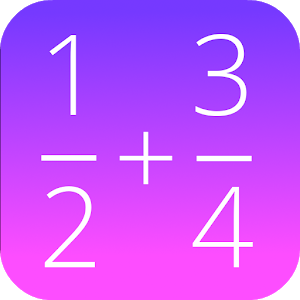Türkçe

Her gün ücretsiz lisanslı Android uygulamaları ve oyunlar sunuyoruz.\$0.99
SÜRESI DOLMUŞ

# Android Giveaway of the Day - Fractions Math Pro

This math app is a fractions calculator with various functions!
\$0.99 SÜRESI DOLMUŞ
Kullanıcı puanı: 6 (100%) 0 (0%)

Bu programın süresi doldu. Fractions Math Pro artık firmadan temin edilebilir.

This calculator is the pro version of 'Fractions Math', and completely without advertisement.

This math app is a fractions calculator with various functions:

- add, subtract, multiply and divide fractions.
- convert fractions in decimals,
- simplify fractions
- compare fractions
- find the lowest common denominator
- fraction trainer, to learn fraction calculations

GK Apps

Education

4.0

279k

Everyone

1.6 and up

## Fractions Math Pro üzerine yorumlar

Thank you for voting!